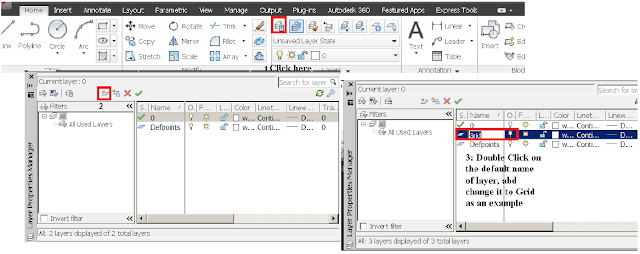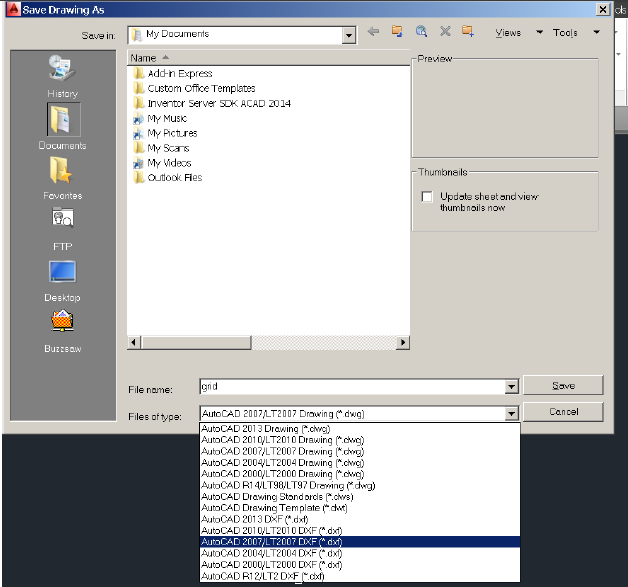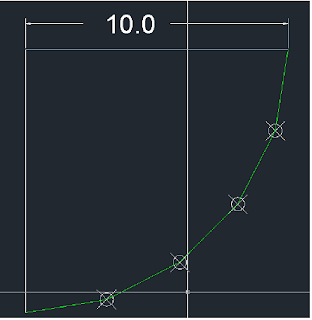Exercise 1: draw the grid system shown in figure 1 and import it to Etabs.[Fig.1]

1- Draw the system in the way you find suitable. If you want to use rectangular option or poly line option, then you have to explode the object you have drawn; Etabs deals with lines only as grid lines; i.e: If you draw rectangular object/poly line then Etabs will not recognize that object as a grid line.

2- Define a new layer name and name it as you want, move the lines drawn previously to this layer. Figure 2 illustrates how to define a new layer through AutoCad (Version 2014)[Fig.2]

3- Move the grid system to the point 0,0. You have the option to move it the you way you find suitable, but here a brief of a way to move your system to the origin: Start the line command, then AutCad will ask you about the first point, type 0,0. Now you have a line that it is starting point is 0,0 , now click at any place in the space and you will have a line it is base point is 0,0, then move your system using move command to that point (figure 3

helps you in understanding this explanation).[Fig.3]

4- Save the file with dxf extension (See figure 4).[Fig.4]

5- Open Etabs, Change units to be in KN and meter, note that you have drawn in meter then you have to create a file in meter.

6- Now do as shown in figure 5.

1
2
3[Fig.5]

7- User now should have as shown in figure 6.[Fig.6]

Importing Curves from AutoCad to Etabs
User may want to move a curve from AutoCad to Etabs, here is the procedure user can follow.
1- User may want to define the curve shown in figure 7.[Fig.7]

2- Use DIVIDE command to divide the curve to number of segments you find approximating your curve, here user may wish to divide the curve into 5 segments (see figure 8 that illustrate divide command).[Fig.8]

3- Using point style option in AutoCad, user may change the style to a shape that help in viewing the points at the curve (a result of divide command) (see figure 9)[Fig.9]

Now user should have as shown in figure 10.[Fig.10]

4- Now Join between points with lines (using line command) (see figure 11).[Fig.11]

5- Delete the curve and keep the lines in between points (see figure 12).[Fig.12]

6- Save the file as a dxf file, then import it typically as done previously with the cartesian system.